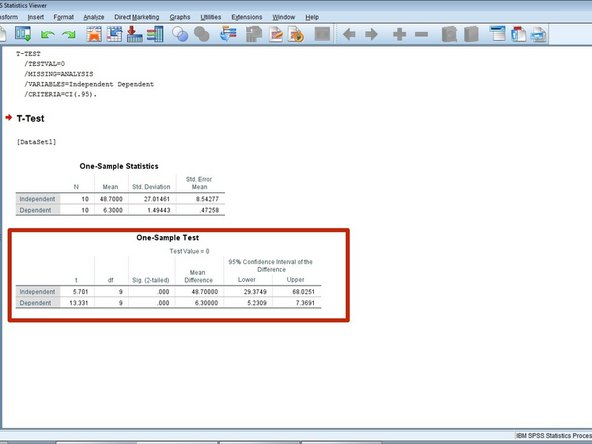Zum Hauptinhalt wechseln

Englisch
Deutsch

## Schritt 13 übersetzen

Schritt 13Understanding the Results Continued
• The second chart is the t-test:

• This chart will be helpful to determine whether the results are statistically significant.

• The first column is the t score of the data.

• The second column is the degrees of freedom.

• The third column is the sig value of the data.

• P-value / sig.: The number that says where on a standard bell curve a value needs to be in order to be statistically significant [ex.: p-value of .05 means that a value must be outside the middle 95% of a bell curve to be statistically significant] (if we use this, we should probably have an image with it)

• The final columns are the ranges inside the confidence intervals.

• Confidence Interval Range: an interval that consist of a range of values that are likely to have the unknown parameter.

Hier Übersetzung einfügen

Hier Übersetzung einfügen

Hier Übersetzung einfügen

Hier Übersetzung einfügen

Hier Übersetzung einfügen

Hier Übersetzung einfügen

Hier Übersetzung einfügen

Hier Übersetzung einfügen

Deine Beiträge sind lizenziert unter der Open-Source Creative Commons Lizenz.